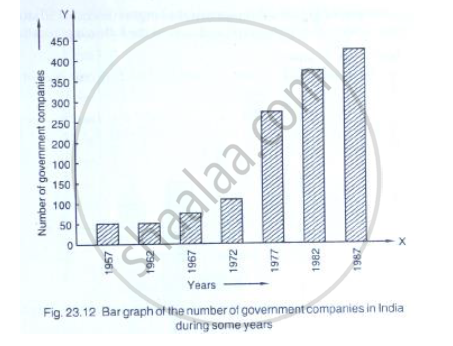# Read the Following Bar Graph and Answer the Following Questions(I) What is the Information Given by the Bar Graph? (Ii) State Each of the Following Whether True Or False. - Mathematics

Read the following bar graph (Fig. 23.12) and answer the following questions:
(i) What is the information given by the bar graph?
(ii) State each of the following whether true or false.
a. The number of government companies in 1957 is that of 1982 is 1 :9.
b. The number of government companies have decreased over the year 1957 to 1983.#### Solution

(i) The given Bar graph represents the number of government companies in india during some years.

(ii) (a) no. of government companies in 1957=50
Number of government companies in 1982=375
∴ The number of government companies in 1957 is that of 1982 = 50/375 = 2/15 ≠ 1/9

So, the given statement is false
(b) The height of the bars increases over the years hence, the statement is false.

Concept: Graphical Representation of Data
Is there an error in this question or solution?

#### APPEARS IN

RD Sharma Mathematics for Class 9
Chapter 23 Graphical Representation of Statistical Data
Exercise 23.1 | Q 8 | Page 10

Share